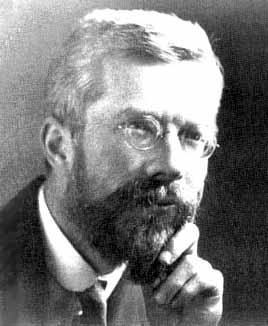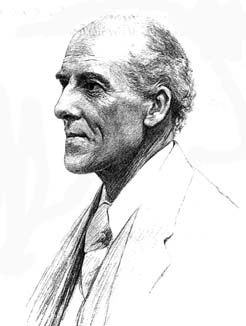## R.A. Fisher (1890-1962)• Revolutionized statistics with the concept of “likelihood,” and maximum likelihood parameter estimation rather than “method of moments.”
• Stated that the “Information” contained in a random sample is inversely related to its variance.
• Wrote the first book on Design-of-Experiments.
• Corrected the inventor of the Chi-square test statistic, introducing the concept of degrees-of-freedom.
• Formalized “Student’s” ideas on the influence of small sample sizes.

## Karl Pearson (1857-1936)• Developed the “Method of Moments,” for estimating statistical model parameters by equating the empirical monents  with the corresponding theoretical ones.
• Chi distance. A precursor and special case of the Mahalanobis distance
• p-value. (Unfortunately, very often misused)
• Suggested the foundations of the statistical hypothesis testing and statistical decision theory
• Invented Pearson’s chi-squared test.
• Suggested rudimentary principal component analysis.